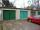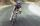# Unit conversion + expression of a variable from formula - math problems

1. PumpsPump that draws water at velocity 3.5 liters per second water from a construction trench take 35 minutes. a) Find out how many minutes the water would run out of the trench pump that draws 7.4 liters of water per second. b) What is the pumping velocity wo
2. Iron sphereIron sphere has weight 100 kg and density ρ = 7600 kg/m3. Calculate the volume, surface and diameter of the sphere.
3. VintnerHow high can vintner fill keg with crushed red grapes if these grapes occupy a volume of 20 percent? Keg is cylindrical with a diameter of the base 1 m and a volume 9.42 hl. Start from the premise that says that fermentation will fill the keg (the number.
4. BMI indexCalculate BMI (body mass index, an index indicating obesity, overweight, normal weight, underweight) man weighing m = 71 kg and height h = 170 cm. Index is calculated according to equation (formula): ? With BMI index is possible to compare people of diff
5. Prism - boxThe base of prism is a rectangle with a side of 7.5 cm and 12.5 cm diagonal. The volume of the prism is V = 0.9 dm3. Calculate the surface of the prism.
6. MoonWe see Moon in the perspective angle 28'. Moon's radius is 1740 km at the time of the full moon. Calculate the mean distance of the Moon from the Earth.Tree perpendicular to the horizontal surface has a shadow 8.32 meters long. At the same time meter rod perpendicular to the horizontal surface has shadow 64 cm long. How tall is tree?
8. PotCalculate the height of 3 liter pot with shape cylinder with a diameter of 10 cm.
9. Aquarium 6How high is the water level in the aquarium with a rectangular base 40cm and 50cm if it is filled 0,65hl of water?
10. PrismThe volume of tetrahedral prism is 2.43 m3. Base of prism is a parallelogram in which a side 2,5dm and height ha = 18cm. Calculate the height of the prism.
11. Tetrahedral prism - rhomboid baseCalculate the area and volume tetrahedral prism that has base rhomboid shape and its dimensions are: a = 12 cm, b = 70 mm, v_a = 6 cm, v_h = 1 dm.
12. TentCalculate how many liters of air will fit in the tent that has a shield in the shape of an isosceles right triangle with legs r = 3 m long the height = 1.5 m and a side length d = 5 m.
13. Water in aquariumThe aquarium cuboid shape with a length of 25 cm and a width of 30 cm is 9 liters of water. Calculate the areas which are wetted with water.
14. LathsThere are two laths in the garage opposite one another: one 2 meters long and the other 3 meters long. They fall against each other and lean against the opposite walls of the garage both laths and touch at a height of 70 cm above the garage floor. How wide
15. Sugar cubesThe glass has 600 ml of tea, which represents 80% of the volume of the glass. If you put twenty regular sugar cubes of 2 cm in the tea, how many ml of tea are poured?
16. PrismCalculate the height of the prism having a surface area 448.88 dm² wherein the base is square with a side of 6.2 dm. What will be its volume in hectoliters?
17. Average speedWhen the bus stops at bus stops driving average speed is 45 km/h. If it did not stop it drive at speed 54 km/h. How many minutes of every hour it spend at stops?
18. Car and cyclistCyclist started from town at speed 18 km/h. After 1 hour 30 min started the car from town and caught up with the cyclist in 50 minutes. How fast was a car?
19. Two cyclistsTwo cyclists started from crossing in the same time. One goes to the north speed 20 km/h, the second eastward at speed 26 km/h. What will be the direct distance cycling 30 minutes from the start?
20. ContainerThe container has a cylindrical shape the base diameter 0.8 m and the area of the base is equal to the area of the wall. How many liters of water can we pour into the container?

Do you have an interesting mathematical word problem that you can't solve it? Enter it, and we can try to solve it.

To this e-mail address, we will reply solution; solved examples are also published here. Please enter the e-mail correctly and check whether you don't have a full mailbox.

Please do not submit problems from current active competitions such as Mathematical Olympiad, correspondence seminars etc...